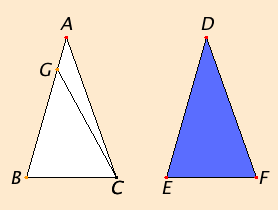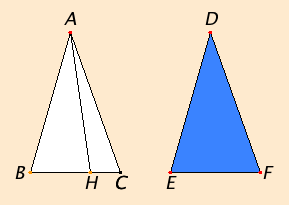# Proposition 26

If two triangles have two angles equal to two angles respectively, and one side equal to one side, namely, either the side adjoining the equal angles, or that opposite one of the equal angles, then the remaining sides equal the remaining sides and the remaining angle equals the remaining angle.

Let ABC and DEF be two triangles having the two angles ABC and BCA equal to the two angles DEF and EFD respectively, namely the angle ABC to the angle DEF, and the angle BCA to the angle EFD, and let them also have one side equal to one side, first that adjoining the equal angles, namely BC equal to EF.

I say that the remaining sides equal the remaining sides respectively, namely AB equals DE and AC equals DF, and the remaining angle equals the remaining angle, namely the angle BAC equals the angle EDF.If AB does not equal DE, then one of them is greater.

Let AB be greater. Make BG equal to DE, and join GC.

Since BG equals DE, and BC equals EF, the two sides GB and BC equal the two sides DE and EF respectively, and the angle GBC equals the angle DEF, therefore the base GC equals the base DF, the triangle GBC equals the triangle DEF, and the remaining angles equal the remaining angles, namely those opposite the equal sides. Therefore the angle GCB equals the angle DFE. But the angle DFE equals the angle ACB by hypothesis. Therefore the angle BCG equals the angle BCA, the less equals the greater, which is impossible.

Therefore AB is not unequal to DE, and therefore equals it.

I.4

But BC also equals EF. Therefore the two sides AB and BC equal the two sides DE and EF respectively, and the angle ABC equals the angle DEF. Therefore the base AC equals the base DF, and the remaining angle BAC equals the remaining angle EDF.

Next, let sides opposite equal angles be equal, as AB equals DE.

I say again that the remaining sides equal the remaining sides, namely AC equals DF and BC equals EF, and further the remaining angle BAC equals the remaining angle EDF.

If BC is unequal to EF, then one of them is greater.Let BC be greater, if possible. Make BH equal to EF, and join AH.

I.4

Since BH equals EF, and AB equals DE, the two sides AB and BH equal the two sides DE and EF respectively, and they contain equal angles, therefore the base AH equals the base DF, the triangle ABH equals the triangle DEF, and the remaining angles equal the remaining angles, namely those opposite the equal sides. Therefore the angle BHA equals the angle EFD.

But the angle EFD equals the angle BCA, therefore, in the triangle AHC, the exterior angle BHA equals the interior and opposite angle BCA, which is impossible.

Therefore BC is not unequal to EF, and therefore equals it.

I.4

But AB also equals DE. Therefore the two sides AB and BC equal the two sides DE and EF respectively, and they contain equal angles. Therefore the base AC equals the base DF, the triangle ABC equals the triangle DEF, and the remaining angle BAC equals the remaining angle EDF.

Therefore if two triangles have two angles equal to two angles respectively, and one side equal to one side, namely, either the side adjoining the equal angles, or that opposite one of the equal angles, then the remaining sides equal the remaining sides and the remaining angle equals the remaining angle.

Q.E.D.

## Guide

There are two statements in this theorem which are different only in their hypotheses. In one, the known side lies between the two angles, in the other, the known side lies opposite one of the angles. If this proposition had come after proposition I.32 which states the sum of the angles in a triangle equals two right angles, then these two hypotheses could have been merged into one, since then if two angles are known, then is the third. But proposition I.32 depends on the parallel postulate I.Post.5, which, it is apparent, Euclid did not want to use unless necessary. Thus, this proposition, I.26, appears where it is with two distinct hypotheses.

#### On congruence theorems

This is the last of Euclid’s congruence theorems for triangles. Euclid’s congruence theorems are I.4 (side-angle-side), I.8 (side-side-side), and this one, I.26 (side and two angles). Calling them congruence theorems is anachronistic, since Euclid did not explicitly use the concept of congruence. We would say that two triangles ABC and DEF are congruent if the angles A, B, and C equal the angles D, E, and F respectively, and the sides AB, BC, and AC equal the sides DE, EF, and DF respectively, and the triangle ABC equals the triangle DEF (by which is meant that they have the same area).

The remaining congruence theorem, side-side-angle, includes some ambiguous cases. Suppose triangles ABC and DEF are such that sides AB and BC are equal to sides DE and EF respectively, and angle A equals angle D. If it is also known that AB is less than or equal to BC, then it follows that the two triangles are congruent. If, however, AB is greater than BC, then the two triangles need not be congruent. Euclid does not include any form of a side-side-angle congruence theorem, but he does prove one special case, side-side-right angle, in the course of the proof of proposition III.14.

Although Euclid does not include a side-side-angle congruence theorem, he does have a side-side-angle similarity theorem, namely proposition VI.7. The analogous congruence theorem could be stated as follows: If two triangles have one angle equal to one angle, two sides adjoining the equal angles equal, namely, one side adjoining the equal angles, and one opposite the equal angles, and the remaining angles either both less or both not less than a right angle, then the remaining side equals the remaining side and the remaining angles equal the remaining angles.

#### Use of Proposition 26

This proposition is used in the proofs of proposition I.34 and several propositions in Books III, IV, XI, XII, and XIII.

As in propositions I.4 and I.8, it appears that the triangles are in the same plane, but, again, that is not necessary. Indeed, this proposition is invoked in proposition XI.35 when two triangles do not lie in the same plane.